# xlf QandA series

## Function arguments and random number series

### QUESTION

WHY DOES MY MONTE CARLO BASED OPTION PRICING FUNCTION SHOW TWO DIFFERENT VALUES IN THE FUNCTION ARGUMENTS DIALOG BOX? (Figure 1)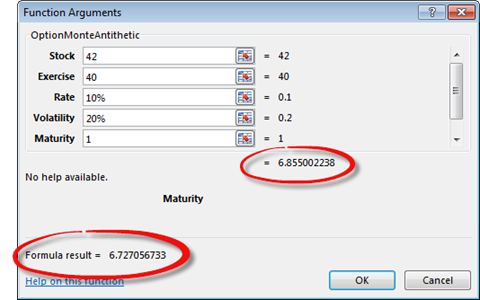Fig 1: The function arguments dialog box - shows two different values for the formula result of the Monte Carlo option pricing formula

###
Answer

The Monte Carlo option pricing procedure uses a random number generator (RNG) to simulate random drawing from the standard normal distribution. This is the epsilon $\varepsilon$ value in the geometric Brownian motion equation for the change in the stock price $\Delta S=\mu S \Delta t+\sigma S \varepsilon \sqrt{\Delta t}$ with rate of return $\mu$ and volatility $\sigma$.

In VBA, $\varepsilon$ can be generated by the expression Application.NormSInv(Rnd()).. Written as Application.Norm_S_Inv(Rnd()) in Excel 2016. In a new session of Excel, ie when Excel has just been opened, it is well known that the VBA RNG always returns the same sequence of random numbers. This feature is useful in answering this question. The code 1 procedure generates the first 10 values of $\varepsilon$.

Code 1: Sub GenEpsilon returns a column vector of 10 $\varepsilon$ variates from the $N(0,1)$ distribution
Sub GenEpsilon()
Dim i As Long
With ActiveCell
.Value = "Iteration"
.Offset(0, 1) = "Random No"
For i = 1 To 10
.Offset(i, 0) = i
.Offset(i, 1) = Application.NormSInv(Rnd())
Next i
End With
End Sub


With output to Excel as shown in figure 2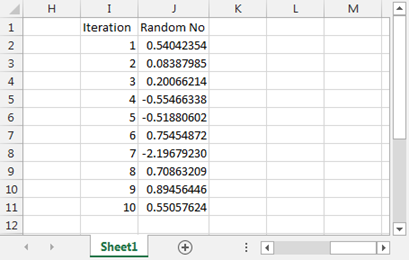Fig 2: The first 10 values - of $\varepsilon$ from the Application.NormSInv(Rnd()) formula in VBA.

Now consider the function procedure in code 2. This procedure is designed to pass the random number sequence in figure 2 through the function arguments dialog box.

Code 2: A function to generate $\varepsilon$ variates
Function TestEpsilon(Num As Double)
TestEpsilon = Application.NormSInv(Rnd()) * Num
End Function


When the TestEpsilon function in code 2 is called in a new session of Excel, it displays the arguments shown in figure 3.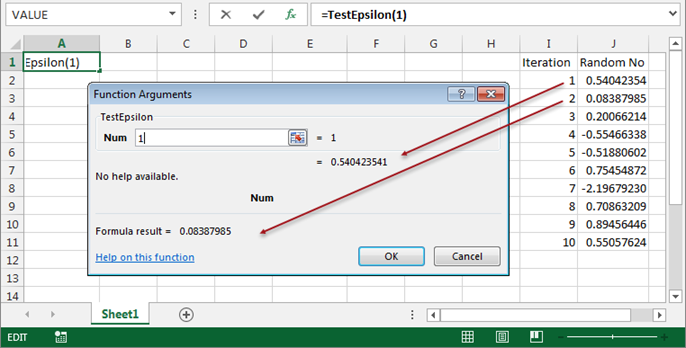Fig 3: The function returns - the first random number (iteration 1) and the formula result is the second random number, iteration 2 in the sequence

Thus, the two numbers in figure 3 are the return values from two consecutive iterations of the RNG. There is an additional iteration between the function return value number and the "Formula result" value. When the OK button is clicked, however, the third iteration, 0.20066214 is returned to cell A1 (figure 4).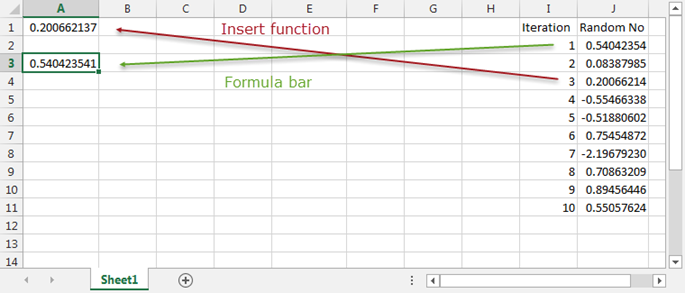Fig 4: The value returned - to the cell via the Insert Function item is the third random number (iteration 3). In contrast the Formula Bar entry is the first iteration in the sequence

Return now to the original Monte Carlo option pricer procedure in figure 1. Suppose that the calling cell has a currency format with 4 decimal places.